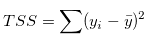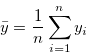# Data Fitting

The aim of data fitting is to estimate the parameters which best describe experimental data (the response values yi).

## Fitting algorithms

QtiPlot uses least-squares algorithms for linear and nonlinear fitting of experimental data. For nonlinear fitting the following iterative algorithms are provided:

## Weighted data fitting

QtiPlot provides several methods in order to calculate the weighting coefficients, wi, for the experimental data. In all cases, for both linear and nonlinear fitting, the least-squares algorithms available in QtiPlot support only Y weights. Even if data sets defined as X error bars are used as weighting coefficients, the fitting algorithms will still treat them as Y weights.

## Goodness-of-Fit Statistics

After performing a data fit operation, a series of fit statistics are displayed in the log window allowing evaluation of the goodness of fit. The default fit statistics to be displayed can be customized via the Fitting page of the Preferences dialog.

Figure 6-3. The results shown in the log window after a data fit operation.The statistic values that QtiPlot can calculate are:

1. N:

the number of response values yi (i.e. data points in the analysed curve/data set).

2. Degrees of Freedom (doF):

defined as the number of response values minus the number of fitted coefficients estimated from these response values: n - p.

3. RSS (Residual Sum of Squares):

is the sum of squares of residuals. This statistic measures the total deviation of the response values from the fit to the response values. It is also called the Sum of Squares due to Error (SSE) or chi-square (Chi^2). A small RSS indicates a tight fit of the model to the data.

4. Chi^2/doF:

The reduced chi-square is obtained by dividing the residual sum of squares (RSS) by the degrees of freedom (doF). Although this is the quantity that is minized during the iterative process, it is typically not a good measure for the goodness of fit. For example, if the y data is multiplied by a scaling factor, the reduced chi-square will be scaled as well.

5. R-square:

is defined as 1 - RSS/TSS, where TSS is the total sum of squares:withbeing the mean of the response values.

In the case of weighted data fitting, the total sum of squares is calculated as:where wi are the weighting coefficients of the response values. This formula uses a correction factor, the mean of the response values being replaced by a weighted mean. Please note that up to release 1.0.0-rc3 QtiPlot used the unweighted mean for the calculation of the total sum of squares.

The R-square statistic measures how successful the fit is in explaining the variation of the data. Put another way, R-square is the square of the correlation between the response values and the predicted response values. It is also called the square of the multiple correlation coefficient and the coefficient of multiple determination.

R-square can take on any value between 0 and 1, with a value closer to 1 indicating that a greater proportion of variance is accounted for by the model. For example, an R-square value of 0.8234 means that the fit explains 82.34% of the total variation in the data about the average.

If you increase the number of fitted coefficients in your model, R-square will increase although the fit may not improve in a practical sense. To avoid this situation, you should use the degrees of freedom adjusted R-square statistic described below.

Note that it is possible to get a negative R-square for equations that do not contain a constant term. Because R-square is defined as the proportion of variance explained by the fit, if the fit is actually worse than just fitting a horizontal line then R-square is negative. In this case, R-square cannot be interpreted as the square of a correlation. Such situations indicate that a constant term should be added to the model.

6. R:

is calculated as the square root of R-square

is defined as 1 - RSS*(n - 1)/(doF*TSS)

The adjusted R-square statistic is generally the best indicator of the fit quality when you compare two models that are nested - that is, a series of models each of which adds additional coefficients to the previous model. The adjusted R-square statistic can take on any value less than or equal to 1, with a value closer to 1 indicating a better fit. Negative values can occur when the model contains terms that do not help to predict the response.

8. RMSE (Root Mean Squared Error):

is calculated as the square root of the reduced Chi-square

RMSE = [Chi2/(n - p)]1/2

This statistic is also known as the fit standard error and the standard error of the regression. It is an estimate of the standard deviation of the random component in the data and is defined as the square root of RSS divided by the degrees of freedom. Just as with RSS, an RMSE value closer to 0 indicates a fit that is more useful for prediction.

The detailed explanations about the meaning of these statistical values were taken from: http://web.maths.unsw.edu.au/~adelle/Garvan/Assays/GoodnessOfFit.html

## Confidence interval for the fit parameters

For each of the p fit parameters, βj, a confidence interval can be calculated using the formulas bellow:

UCL = βj + t(1-α/2,n-p)εj

LCL = βj - t(1-α/2,n-p)εj

where UCL is the Upper Confidence Limit, LCL is the Lower Confidence Limit, t(1-α/2,n-p) is the 100(1 - α/2) percentage point of Student's t distribution on n - p degrees of freedom, n is the number of data points and εj is the standard error for the j-th parameter, calculated from the corresponding diagonal element of the covariance matrix Cov(j, j) as explained in the Reported errors section of the chapter dedicated to the fit wizard.

## Confidence bands

In the case of unweighted data fitting, the confidence interval around a predicted response Ŷi at a particular abscissa Xi is calculated as:

Yi = Ŷi Ý t(1-α/2,n-p)S[1/n + (Xi - Xm)2/Sxx]1/2

where t(1-α/2,n-p) is the 100(1 - α/2) percentage point of Student's t distribution on n - p degrees of freedom, n is the number of data points, S is the Root Mean Squared Error (RMSE), Xm = ∑Xi/n is the mean of the abscissas of the data points and Sxx = ∑(Xi - Xm)2.

For weighted data fitting, the prediction interval around a predicted response Ŷi at a particular abscissa Xi is calculated as:

Yi = Ŷi Ý t(1-α/2,n-p)Sw[1/∑wi + (Xi - Xmw)2/Sxxw]1/2

where Sw is the weighted Root Mean Squared Error (RMSE), Sxxw = ∑wi(Xi - Xmw)2 and the weighted mean of the abscissas is defined as Xmw = ∑wiXi/∑wi.

## Prediction bands

In the case of unweighted data fitting, the prediction interval around a predicted response Ŷi at a particular abscissa Xi is calculated as:

Yi = Ŷi Ý t(1-α/2,n-p)S[1 + 1/n + (Xi - Xm)2/Sxx]1/2

where t(1-α/2,n-p) is the 100(1 - α/2) percentage point of Student's t distribution on n - p degrees of freedom, n is the number of data points, S is the Root Mean Squared Error (RMSE), Xm = ∑Xi/n is the mean of the abscissas of the data points and Sxx = ∑(Xi - Xm)2.

For weighted data fitting, the prediction interval around a predicted response Ŷi at a particular abscissa Xi is calculated as:

Yi = Ŷi Ý t(1-α/2,n-p)Sw[1 + 1/∑wi + (Xi - Xmw)2/Sxxw]1/2

where Sw is the weighted Root Mean Squared Error (RMSE), Sxxw = ∑wi(Xi - Xmw)2 and the weighted mean of the abscissas is defined as Xmw = ∑wiXi/∑wi.

For more explanations about the formulas for the confidence and prediction intervals, please read the article Weighted Least-Squares Approach To Calculating Limits of Detection and Quantification by Modeling Variability as a Function of Concentration by Michael E. Zorn, Robert D. Gibbons, and William C. Sonzogni.